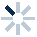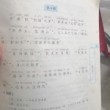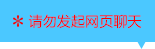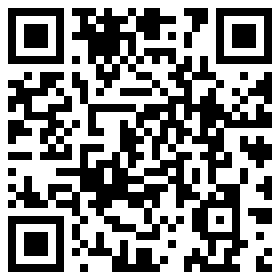| | |

||
APP端下载AndroidiPhone
|
￥39

|
571人点赞
210985人已学习
|

3天无理由退款

3天无理由退款：退款将以超级币形式退至您的超级课堂学习账户，便于您重新选购其他课程。恶意退款将被冻结账号。

• 1、关于零点的定义有三点需要注意：(1)方程$f(x)=0$有实数根等价于函数$y=f(x)$的图象与$x$轴有交点等价于函数$y=f(x)$有零点；(2)零点不是点，而是实数；(3)方程的根的个数$\geq$函数的零点的个数，只有当方程没有重根时，它们才相等
2、 要判断$x_{0}$是否是$f(x)$的零点，只要验证$f(x_{0})=0$是否成立即可
• 1、​用代数法求零点或零点个数，其实就是解方程
2、 对于含有绝对值的方程和二次项含有参数的二次方程，都要注意分类讨论，而在求参数范围时，可以采用参变分离的方法
• 1、​用图象法求零点个数时，函数图象与$x$轴交点的个数，就是零点的个数
2、 形如$F(x)=f(x)-g(x)$的函数，零点就是函数$y=f(x)$的图象与函数$y=g(x)$的图象交点的横坐标
3、 形如$f(x)=g(x)-C$，$C$为常数的函数，零点就是函数$y=g(x)$的图象与$y=C$的图象交点的横坐标
• 1、介绍了零点的逆用，就是通过零点个数来确定图象的大致位置，从而确定解析式中参数的取值范围
• 1、掌握函数零点的判定定理，判定$f(x)$在区间$(a'b)$上存在零点，只需要满足两条：一，图象连续；二，端点函数值异号，即$f(a)·f(b)$小于$0$
• 1、判断$f(x)$在区间$(a,b)$上存在唯一零点，只需要满足三条：一，图象连续；二、函数单调；三、端点函数值异号
2、 且对于连续的单调函数来说，$f(a)·f(b)$小于$0$与$f(x)$在$(a,b)$内存在一个零点，是完全等价的
• 1、掌握第一种零点分布：在某个点的两侧或同侧
2、 两侧时，限定$a\cdot f(r)＜0$即可。同侧时，可以通过三个不等式构成的不等式组来限定，分别用到了端点函数值、对称轴和判别式
• 1、​掌握第二种零点分布：两个零点分别位于$(p,q)$与$(m,n)$内$(p＜q\leq m＜n)$
2、 可以通过这四个由开口方向和端点函数值构成的不等式组来限定
• 1、掌握第三种零点分布：$f(x)$在区间$(p,q)$内有一个或两个零点
2、 两个零点时，也要通过端点函数值、对称轴和判别式这三点，构成四个不等式来限定
3、 一个零点时，要先按相切和相交分类。相切时，$\Delta =0$， 然后求出切点，即零点，验证它是否在指定开区间内。相交时，又可以细分为两类：第一类：一个零点在开区间内，另一零点在开区间外。通过$f(p)\cdot f(q)＜0$限定即可；第二类：一个零点在开区间内，另一零点恰好是开区间的端点
• 1、灵活运用前三节课学习的零点知识，解决一道二次函数的三种零点分布在综合难题
• 1、二分法是通过取区间中点，看正负，不断缩小区间范围，无限逼近零点的一种求零点近似值的方法
2、 要注意“精确度”这种表述的区别，它限制了区间范围的大小
• 1、了解二分法的适用条件。只有变号零点才能用二分法，不变号零点是不能的
2、 还要注意“精确到”这种表述的意义,“精确到”规定了数据的精确度
• 1、对于二分法求形如$\sqrt{a}$近似值的题目，都可以先构造出形如$f(x)=x^{2}-a$的函数，然后用二分法找零点近似值，也就是$\sqrt{a}$的近似值
• 1、学习二分法的第二种巧妙应用：求二分次数
2、 求二分次数，有公式$(b-a)·\dfrac{1}{2^{n}}＜\varepsilon$ 。其中$b-a$代表区间长度，在应用题中，一般代表测量总数，测量总长等数据。$\varepsilon$代表精确度，在应用题中，一般代表精确范围，如果要找出个体，则$\varepsilon =1$，且不等号用$\leq$号
• 函数的应用综合练习

• 1超级学员856617
• 2小Eva
• 3y8to
• 4进击的Er
• 5学员060
• 6呃呃呃呃呃
• 7uKN95
• 8超级学员4228048
• 9真无聊啊
• 10超级学员4351556196446人在学
￥ 78 ￥ 78127120人在学
￥ 78 ￥ 78219511人在学
￥ 0 ￥ 0126303人在学
￥ 72 ￥ 72

• 0
• 点击分享有好礼
••app端下载关注微信号